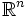# Negatively curved manifold

Thiss article defines a property that can be evaluated for a differential manifold, invariant under diffeomorphisms
View other properties of differential manifolds

## Definition

### Symbol-free definition

A differential manifold$M$ is said to be negatively curved if it can be equipped with a Riemannian metric with negative sectional curvature.

## Facts

The Cartan-Hadamard theorem states that the universal cover of any negatively curved manfiold is diffeomorphic to Euclidean space (viz$\mathbb{R}^n$).